# Underwater Cyclops

It can give you a controlling device that can helps you to build an underwater robot communicating via Ethernet cable.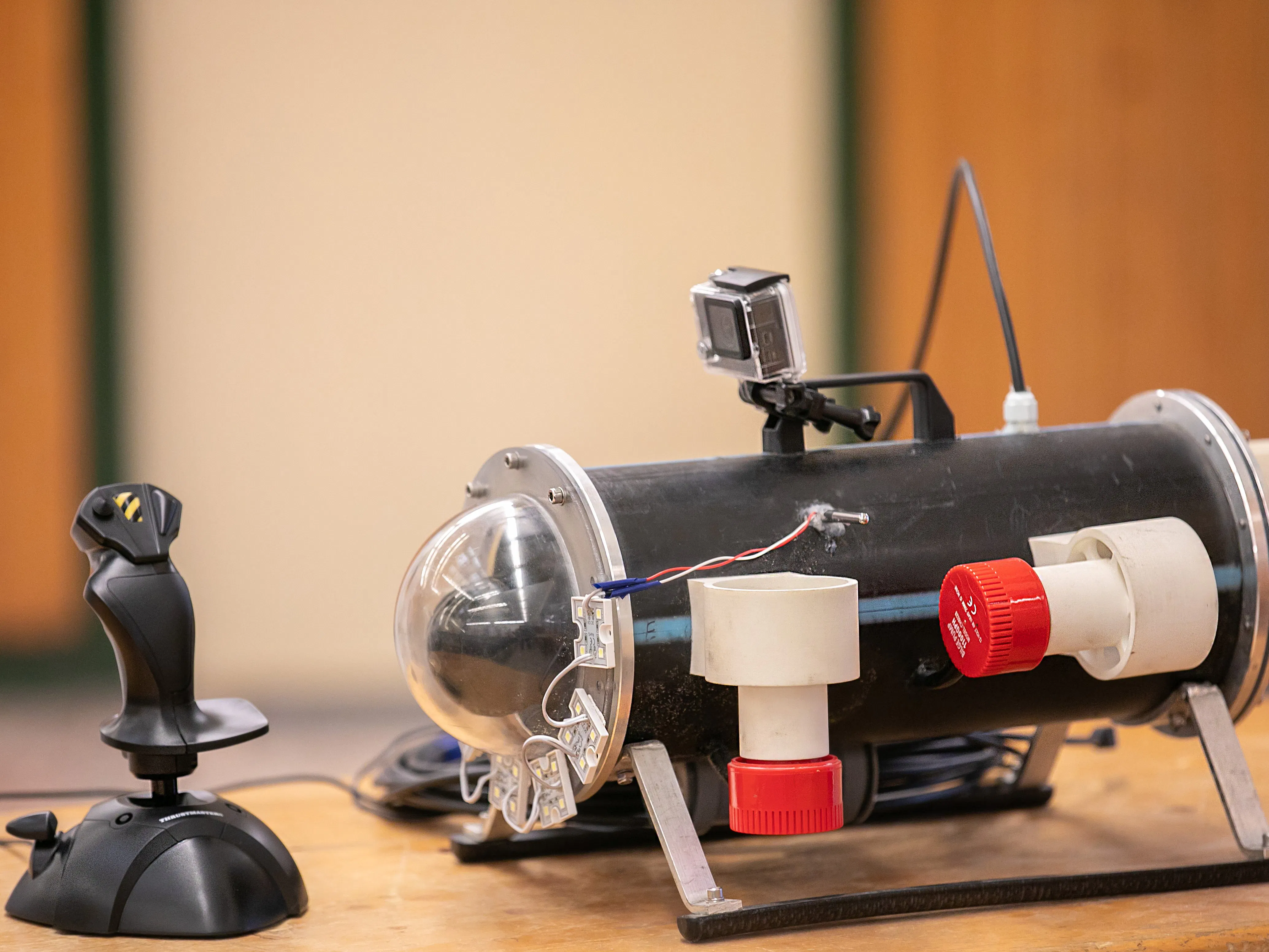## Things used in this project

### Hardware components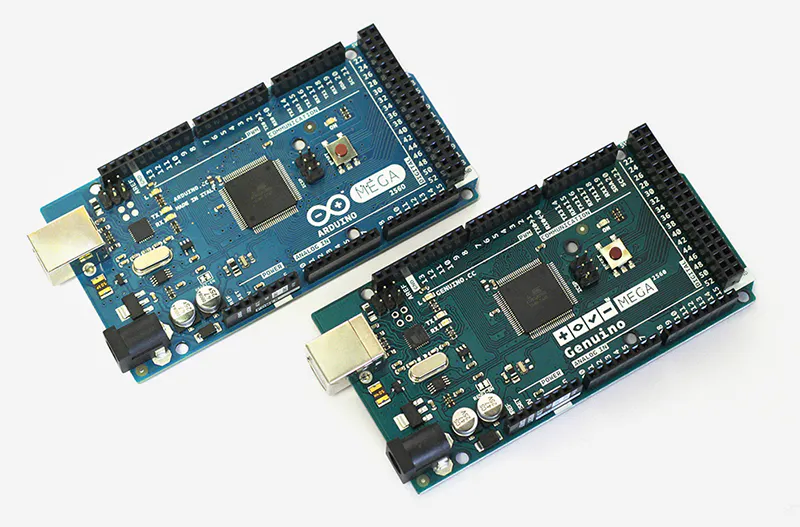Arduino Mega 2560 & Genuino Mega 2560
×1
 DS18B20 temperature sensor
×1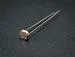Photo resistor
×1Jumper wires (generic)
×1

## Schematics

### DS18B20 temperature sensor example circuit

You can use another input pin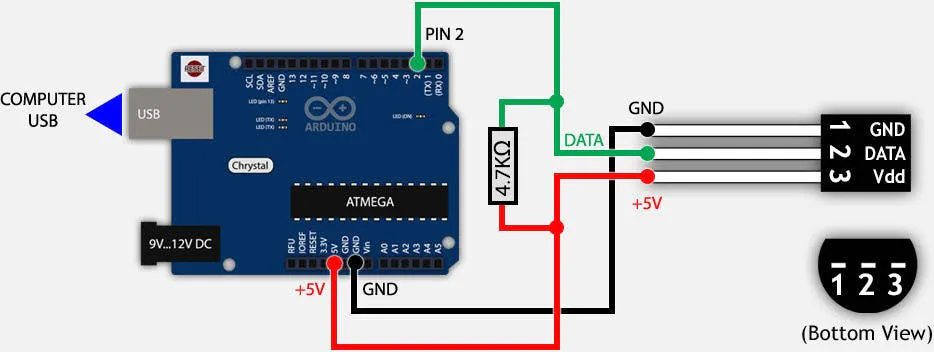### Photo resistor example circuit

You have to use a 10k resistor and you can choose another input pin.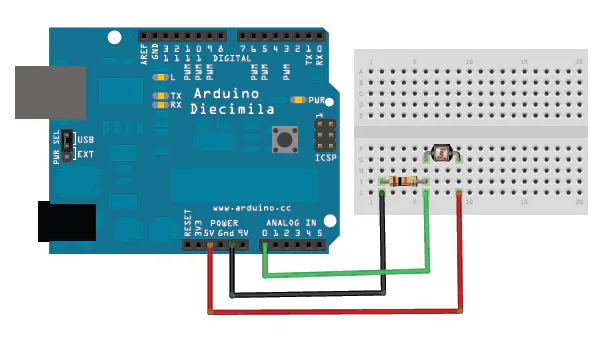### Splitting UTP cable## Code

### Underwater ROV code

Arduino
It's an open source code, you can modify this, or cut useful lines.
```#include <OneWire.h>
#include <DallasTemperature.h>
#include <SPI.h>
#include <Ethernet.h>
#include <EthernetUdp.h>

#define ONE_WIRE_BUS 2
OneWire oneWire(ONE_WIRE_BUS);
DallasTemperature sensors(&oneWire);

byte mac[] = {
0x90, 0xA2, 0xDA, 0x0D, 0x7F, 0xFC
};

unsigned int localPort = 8877;

int fenymennyiseg=A1;
char datain[UDP_TX_PACKET_MAX_SIZE];
float temperature;

EthernetUDP Udp;

int motorA1=39;
int motorA2=41;
int speedA=11; //FEL LE DUPLA MOTOROK
int motorB1=45;
int motorB2=43;
int speedB=9; //FEL-LE MOTOR
int motorC1=49;
int motorC2=47;
int speedC=5; // BAL ELRE HTRA MOZGAT
int motorD1=53;
int motorD2=31;
int speedD=3; //JOBB ELRE HTRA MOZGAT
int LEDEK = 8;
char ertek;
int oldButtonState = LOW;
int regiallas = LOW;
int x=0;
int y=0;

int fenylampa=7;

int sdcs=4;
int ethcs=10;
int valtgomb=0;

void setup()
{

Ethernet.begin(mac, ip);
Udp.begin(localPort);

Serial.begin(9600);
sensors.begin();
pinMode(motorA1,OUTPUT);
pinMode(motorA2,OUTPUT);
pinMode(speedA,OUTPUT);
pinMode(motorB1,OUTPUT);
pinMode(motorB2,OUTPUT);
pinMode(speedB,OUTPUT);
pinMode(motorC1,OUTPUT);
pinMode(motorC2,OUTPUT);
pinMode(speedC,OUTPUT);
pinMode(motorD1,OUTPUT);
pinMode(motorD2,OUTPUT);
pinMode(speedD,OUTPUT);
pinMode(LEDEK, OUTPUT);
pinMode(sdcs, OUTPUT);
digitalWrite(sdcs, HIGH);
pinMode(ethcs, OUTPUT);
digitalWrite(ethcs, LOW);
digitalWrite(LEDEK,HIGH);
pinMode(fenylampa,OUTPUT);
digitalWrite(fenylampa,HIGH);
digitalWrite(speedC,LOW);

}

void loop()
{
String valasz= String("ack ");
String pont= String("\n");
sensors.requestTemperatures();
temperature=sensors.getTempCByIndex(0);
String homerseklet=String(temperature);

String Buffer=fenyallas+pont+valasz+pont+homerseklet;
char cBuffer[Buffer.length()];
Buffer.toCharArray(cBuffer, Buffer.length());
Serial.println(cBuffer);

int packetSize = Udp.parsePacket();
if (packetSize)
{

Serial.println("Contents:");
for(int i = 0; i<10;i+= 2)
{
Serial.print( *(int*)(&ertek[i]));
Serial.print(",");
}
Serial.print("\n");

//------------------------------------------------------------------------------------
if( *(int*)(&ertek) <=-100 && valtgomb!=1)//ELORE-HATRA y tengely
{
analogWrite(speedC, abs(*(int*)(&ertek)));
analogWrite(speedD, abs(*(int*)(&ertek)));
digitalWrite(motorC1, HIGH);
digitalWrite(motorC2, LOW);
digitalWrite(motorD1, LOW);
digitalWrite(motorD2, HIGH);

}
else
if(*(int*)(&ertek) >=100 && valtgomb!=1)
{
analogWrite(speedC, *(int*)(&ertek));
analogWrite(speedD, *(int*)(&ertek));

digitalWrite(motorC1, LOW);
digitalWrite(motorC2, HIGH);
digitalWrite(motorD1, HIGH);
digitalWrite(motorD2, LOW);

}
else
if(*(int*)(&ertek) < 50 && *(int*)(&ertek) >-50 && valtgomb!=1)
{
analogWrite(speedC, 0);
analogWrite(speedD, 0);
}
//------------------------------------------------------------------------------------
if( *(int*)(&ertek) >=50 && valtgomb!=1)//FEL-LE X tengely
{
analogWrite(speedA, *(int*)(&ertek));
analogWrite(speedB, *(int*)(&ertek));
digitalWrite(motorA1, HIGH);
digitalWrite(motorA2, LOW);
digitalWrite(motorB1, HIGH);
digitalWrite(motorB2, LOW);
}
else
if(*(int*)(&ertek) <=-50 && valtgomb!=1)
{
analogWrite(speedA, abs(*(int*)(&ertek)));
analogWrite(speedB, abs(*(int*)(&ertek)));
digitalWrite(motorA1, LOW);
digitalWrite(motorA2, HIGH);
digitalWrite(motorB1, LOW);
digitalWrite(motorB2, HIGH);
}
else
if(*(int*)(&ertek) < 50 && *(int*)(&ertek) >-50 && valtgomb!=1)
{

analogWrite(speedA, 0);
analogWrite(speedB, 0);
}
//------------------------------------------------------------------------------------ledek be/ki

int newButtonState = *(int*)(&ertek);

if (newButtonState == HIGH && oldButtonState == LOW)
{

if (x == 0) {
// Toggle on
digitalWrite(LEDEK, HIGH);
x = 1;

} else {
// Toggle off
digitalWrite(LEDEK, LOW);
x = 0;
}
}

oldButtonState = newButtonState;
//------------------------------------------------------------------------------------//fenymennyisegmero KI/BE

int gomballas = *(int*)(&ertek);

if (gomballas == HIGH && regiallas == LOW)
{

if (y == 0)
{
// Toggle on
digitalWrite(fenylampa, HIGH);
y = 1;

} else {
// Toggle off
digitalWrite(fenylampa, LOW);
y = 0;
}
}

regiallas = gomballas;

//------------------------------------------------------------------------------------
valtgomb=*(int*)(&ertek);
if(valtgomb==1)
{
if( *(int*)(&ertek) >=50 && valtgomb==1)//fordulas
{
analogWrite(speedC, *(int*)(&ertek));
analogWrite(speedD, *(int*)(&ertek));
digitalWrite(motorC1, HIGH);
digitalWrite(motorC2, LOW);
digitalWrite(motorD1, HIGH);
digitalWrite(motorD2, LOW);
}
else
if(*(int*)(&ertek) <=-50 && valtgomb==1)
{
analogWrite(speedC, abs(*(int*)(&ertek)));
analogWrite(speedD, abs(*(int*)(&ertek)));
digitalWrite(motorC1, HIGH);
digitalWrite(motorC2, LOW);
digitalWrite(motorD1, HIGH);
digitalWrite(motorD2, LOW);
}
else
if(*(int*)(&ertek) < 50 && *(int*)(&ertek) >-50 && valtgomb==1)
{
analogWrite(speedC, 0);
analogWrite(speedD, 0);
}

}

//------------------------------------------------------------------------------------
Udp.beginPacket(Udp.remoteIP(), Udp.remotePort());
Udp.write(cBuffer);
Udp.endPacket();

}
else
{
digitalWrite(motorA1,LOW);
digitalWrite(motorA2,LOW);
digitalWrite(motorB1,LOW);
digitalWrite(motorB2,LOW);
digitalWrite(motorC1,LOW);
digitalWrite(motorC2,LOW);
digitalWrite(motorD1,LOW);
digitalWrite(motorD2,LOW);
}
delay(50);
}
```

### Python code

Python
```import socket
import pygame
import struct
import time
import datetime as dt
dt.datetime.now()

UDP_IP = '192.168.1.102'
UDP_PORT = 8877
sock = socket.socket(socket.AF_INET, socket.SOCK_DGRAM)
sock.settimeout(2)

pygame.init()

pygame.joystick.init()

joy_count = pygame.joystick.get_count()

if joy_count < 1 : print ("Nincs joystick csatlakoztatva.")
else :
fenymennyiseg=open("feny.csv","w")
fenymennyiseg.write("DATUM;FENYMENNY\n")

while True :
joystick = pygame.joystick.Joystick(0)
joystick.init()

pygame.event.pump()

y_tengely = int(joystick.get_axis(1) * 255)
x_tengely = int(joystick.get_axis(0) * 255)
gomb = int(joystick.get_button(1))
valtgomb = int(joystick.get_button(3))
fenygomb = int(joystick.get_button(2))

sock.sendto(struct.pack("hhhhh", x_tengely, y_tengely, gomb, valtgomb, fenygomb), (UDP_IP, UDP_PORT))

if (int(joystick.get_button(2))==1) :
fenymennyiseg=open("feny.csv","a")
time.sleep(0.1)
fenymennyiseg.close()

print ("Nincs valasz")
break

```

## Credits

### Bankirobot

0 projects • 7 followers